# I.E. Irodov Solutions on The Fundamental Equation of Dynamics

Students can find the solutions of Problems In General Physics I.E. Irodov - The Fundamental Equation of Dynamics on this page. The Fundamental Equation of Dynamics is an important part of Physical Fundamentals of Mechanics. The branch of Physics which deals with the study of motion when subjected to forces or displacements and the subsequent effects of the bodies on their environment is called mechanics. The important topics of the Fundamental Equation of Dynamics are the fundamental equation of dynamics of a mass point (Newton's second law), the same equation expressed in projections on the tangent and the normal of the point's trajectory, the equation of dynamics of a point in the non-inertial reference frame, etc.

Learning these solutions will help students to score more marks in JEE exam and board exams. Students can improve their speed and accuracy by revising these solutions.

### I.E. Irodov Solutions on The Fundamental Equation of Dynamics

1. An aerostat of mass m starts coming down with a constant acceleration w. Determine the ballast mass to be dumped for the aerostat to reach the upward acceleration of the same magnitude. The air drag is to be neglected.

Solution:

1. Let R be the constant upward thurst on the aerostat of mass m, coming down with a constant acceleration w. Applying Newton’s second law of motion for the aerostat in projection form

Fy = mwy

mg - R = mw ...(1)

Now, if ∆m be the mass, to be dumped, then using the Eq. Fy = mwy

R - (m - ∆m)g = (m - ∆m)w ..(2)

From Eqn. (1) and (2), we get ∆m = 2mw/(g + w)

2. In the arrangement of Fig. the masses mo, m1, and m2 of bodies are equal, the masses of the pulley and the threads are negligible, and there is no friction in the pulley. Find the acceleration w with which the body mo comes down, and the tension of the thread binding together the bodies m1 and m2, if the coefficient of friction between these bodies and the horizontal surface is equal to k. Consider possible cases.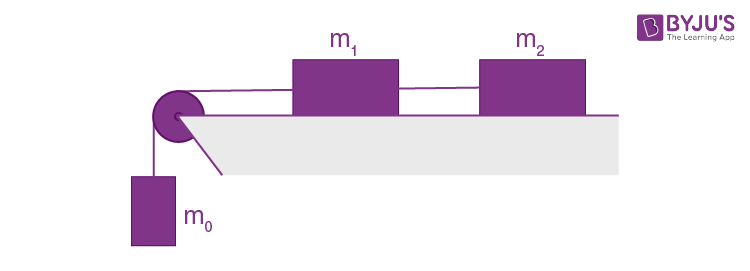Solution:

1. Let us write the fundamental equation of dynamics for all the three blocks in terms of projections, having taken the positive direction of x and y axes as shown in Fig; and using the fact that kinematical relation between the accelerations is such that the blocks move with same value of acceleration (say w)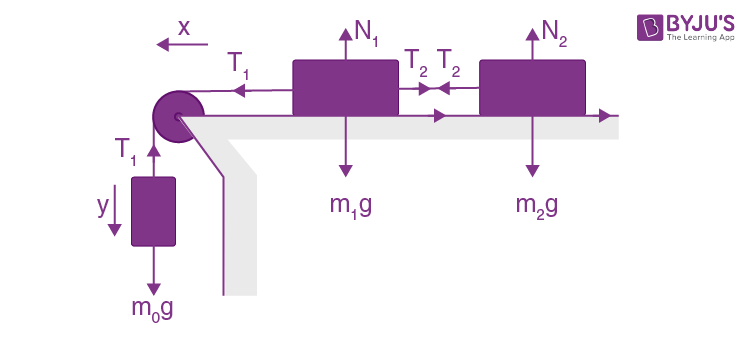m0g - T1 = m0w (1)

T1 - T2 - km1g = m1w (2)

And T2 - km2g = m2w (3)

The simultaneous solution of equations (1), (2) and (3) yields

w = g(m0 - k(m1 + m2))/(m0 + m1 + m2)

And T2 = (1 + k)m0 m2g/(m0 + m1 + m2)

As the block m0 moves down with acceleration w, so in vector form

$\vec{w}=\frac{(m_{0}-k(m_{1}+m_{2}))\vec{g}}{m_{0}+m_{1}+m_{2}}$

3. Two touching bars 1 and 2 are placed on an inclined plane forming an angle α with the horizontal (Fig. 1.10). The masses of the bars are equal to m1 and m2, and the coefficients of friction between the inclined plane and these bars are equal to k1 and k2 respectively, with k1 > k2. Find: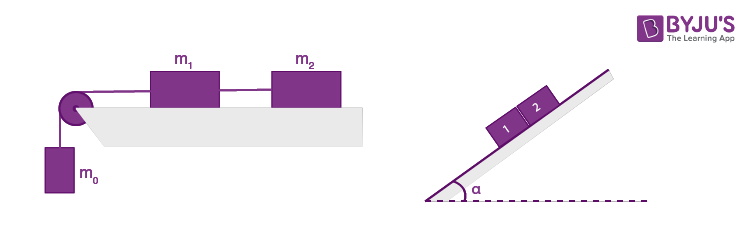1. (a) the force of interaction of the bars in the process of motion;

2. (b) the minimum value of the angle α at which the bars start sliding down.

Solution:

1. Let us indicate the positive direction of x-axis along the incline (Fig.). Figures show the force diagram for the blocks. Let, R be the force of interaction between the bars and they are obviously sliding down with the same constant acceleration w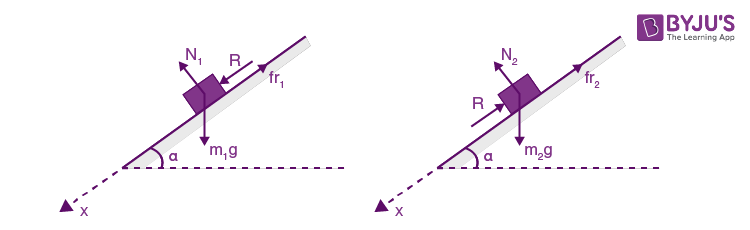Newton’s second law of motion in projection form along x-axis for the blocks gives :

m1g sin α - k1m1 g cos α + R = m1w …(1)

m2g sin α - R - k2m2 g cos α = m2w …(2)

Solving Eqs. (1) and (2) simultaneously, we get

w = g sin α - g cos α(k1m1 + k2m2)/(m1 + m2) and

R = m1m2(k1- k2)g cos α/(m1 + m2) ..(3)

(b) when the blocks just slide down the plane, w = 0 , so from Eqn. (3)

g sin α - g cos α (k1m1 + k2m2)/(m1 + m2) = 0

Or (m1 + m2)sin α = (k1m1 + k2m2)cos α

Hence tan α = (k1m1 + k2m2)/(m1 + m2)

4. A small body was launched up an inclined plane set at an angle α = 150 against the horizontal. Find the coefficient of friction, if the time of the ascent of the body is η = 2.0 times less than the time of its descent.

Solution:

1. Case 1. When the body is launched up :

Let k be the coefficient of friction, u the velocity of projection and l the distance traversed along the incline. Retarding force on the block = mg sin α + k mg cos α

and hence the retardation = g sin α + kg cos α .

Using the equation of particle kinematics along the incline,

0 = u2 -2 (g sin α + kg cos α) l

Case 2. When the block comes downward, the net force on the body = mg sin α - km g cos α and hence its acceleration = g sin α - kg cos α

Let, t be the time required then

l = ½ (g sin α - kg cos α)t’2 ..(4)

From Eqns (3) and (4)

t2/t’2 = (sin α - k cos α)/(sin α + k cos α)

But t/t’= 1/η (according to question)

Hence on solving we get

k = (η2 - 1)/(η2 + 1) tan α = 0.16

5. The following parameters of the arrangement of Fig. 1.11 are available: the angle α which the inclined plane forms with the horizontal, and the coefficient of friction k between the body m1 and the inclined plane. The masses of the pulley and the threads, as well as the friction in the pulley, are negligible. Assuming both bodies to be motionless at the initial moment, find the mass ratio m2/m1 at which the body m2

1. (a) starts coming down;

2. (b) starts going up; (c) is at rest.

Solution:

1. At the initial moment, obviously, the tension in the thread connecting m1 and m2 equals the weight of m2.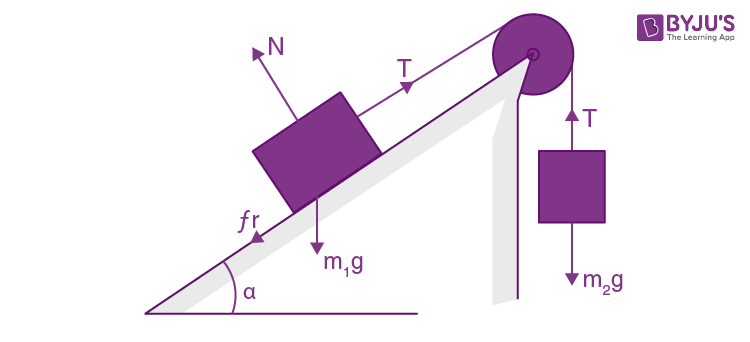(a) For the block m2 to come down or the block m1 to go up, the conditions is

m2g - T ≥ 0 and T - m1g sin α - fr ≥ 0

where T is tension and fr is friction which in the limiting case equals km1g cosα. Then

m2g - m1 sin α > km1 g cos α

Or m2/m1 > (k cos α + sin α)

(b) Similarly in the case

m1g sin α - m2g > frlim

Or m1g sin α - m2g > km1g cos α

Or m2/m1 < sin α - k cos α

(c) For this case, neither kind of motion is possible, and fr need not be limiting.

Hence, (k cos α + sin α)> m2/m1 > (sin α - k cos α)

6. The inclined plane of Fig. 1.11 forms an angle α = 300 with the horizontal. The mass ratio m2/m1 = η = 2/3. The coefficient of friction between the body m1 and the inclined plane is equal to k = 0.10. The masses of the pulley and the threads are negligible. Find the magnitude and the direction of acceleration of the body m2 when the formerly stationary system of masses starts moving.

Solution:

1. From the conditions, obtained in the previous problem, first, we will check whether the mass m2 goes up or down. Here, m2/ m1 = η > sin α + k cos α , (substituting the values). Hence the mass m2 will come down with an acceleration (say w). From the free body diagram of previous problem,

m2 - g -T = m2w (1)

And T - m1g sin α - km1g cos α = m1w (2)

Adding (1) and (2), we get

m2g - m1g sin α - km1g cos α = (m1 + m2)w

w = (m2/m1 - sin α - k cos α)g/(1 + m2/m1)

= (η - sin α - k cos α)g/(1+η)

Substituting all the values, w = 0.048 g ≈ 0.05 g

As m2 moves down with acceleration of magnitude w = 0.05 g > 0, thus in vector form acceleration of m2:

$\vec{w}=\frac{\eta -\sin \alpha -k\cos \alpha }{1+\eta }\vec{g}$

= $0.05\vec{g}$

7. A plank of mass m1 with a bar of mass m2 placed on it lies on a smooth horizontal plane. A horizontal force growing with time t as F = at (a is constant) is applied to the bar. Find how the accelerations of the plank w1 and of the bar w2 depend on t, if the coefficient of friction between the plank and the bar is equal to k. Draw the approximate plots of these dependences.

Solution:

1. Let us write the Newton’s second law in projection form along the positive x-axis for the plank and the bar fr = m1w1 , fr = m2v2

At the initial moment, fr represents the static friction, and as the force F grows so does the friction force fr, but up to its limiting value.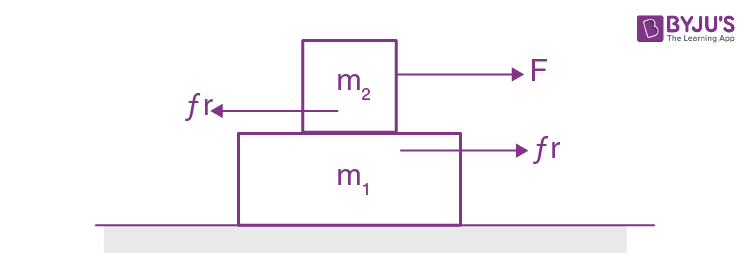I.e. fr = frs(max) = kN = km2g

Unless this value is reached, both bodies move as a single body with equal acceleration. But as soon as the force fr reaches the limit, the bar starts sliding over the plank i.e. w1 ≥ w2.

Substituting here the values of w1 and w2 taken from Eq. (1) and taking into account that

fr = km2g, we obtain, (at - km2g)/m2 ≥ km2g/m1, where the sign = corresponds to the moment t = t0 (say)

Hence t0 = kgm2 (m1 + m2)/am1

If t ≤ t0, then w1 = km2g/m2 (constant) and w2 = (at - km2g)/m2

On this basis w1 (r) and w2 (t), plots are as shown in the figure of answer sheet.

8. A small body A starts sliding down from the top of a wedge (Fig. 1.12) whose base is equal to l = 2.10 m. The coefficient of friction between the body and the wedge surface is k = 0.140. At what value of the angle α will the time of sliding be the least? What will it be equal to?

Solution:

1. Let us designate the x-axis (Fig.) and apply Fx = mwx for body A:

mg sin α - kmg cos α = mw

Or w = g sin α - kg cos α

Now from kinematical equation:

l sec α = 0 + (½)wt2

Or t = √(2l sec α/(sin α - k cos α)g)

= √(2l/(sin 2α/2 - k cos2 α)g)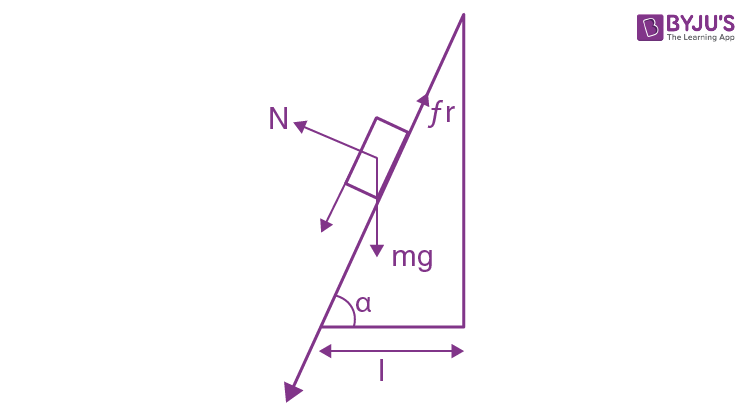Using Eq. (1)

For tmin d(sin 2α/2 - k cos2α)/dα = 0

i.e. 2 cos 2α/2 +2k cos α sin α = 0

Or tan 2α = -1/k => α = 490

and putting the values of α , k and l in Eq . (2) we get tmin = 1s.

9. A bar of mass m is pulled by means of a thread up an inclined plane forming an angle α with the horizontal (Fig. 1.13). The coefficient of friction is equal to k. Find the angle β which the thread must form with the inclined plane for the tension of the thread to be minimum. What is it equal to?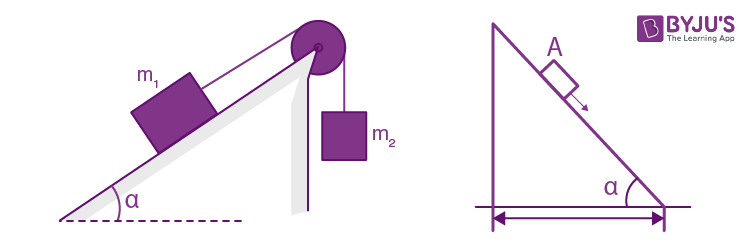Solution:

1. Let us fix the x-y coordinate system to the wedge, taking the x-axis up, along the incline, and the y-axis perpendicular to it (Fig.). Now, we draw the free body diagram for the bar.

Let us apply Newton’s second law in projection form along x and y axis for the bar :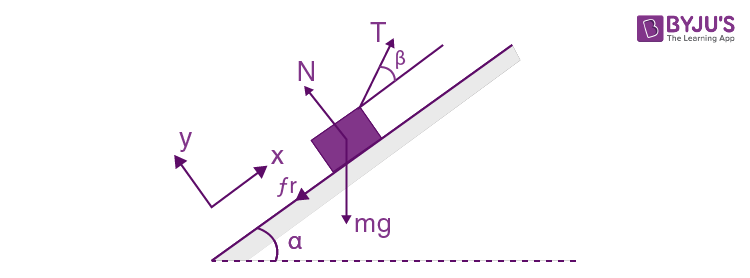T cos β - mg sin α - fr = 0 (1)

T sin β + N - mg cos α = 0

Or N = mg cos α - T sin β (2)

But fr = kN and using (2) in (1), we get

T = mg sin α + kmg cos α/(cos β + k sin β) (3)

For Tmin the value of (cos β + k sin β) should be maximum.

So, d(cos β + k sin β)/dβ = 0 or tan β = k

Putting this value of β in Eq.(3) we get,

Tmin = mg (sin α + k cos α)/1/√(1 + k2) + k2/√(1+k2)

= mg (sin α + k cos α)/√(1+k2)

10. At the moment t = 0 the force F = at is applied to a small body of mass m resting on a smooth horizontal plane (a is a constant). The permanent direction of this force forms an angle α with the horizontal (Fig. 1.14). Find: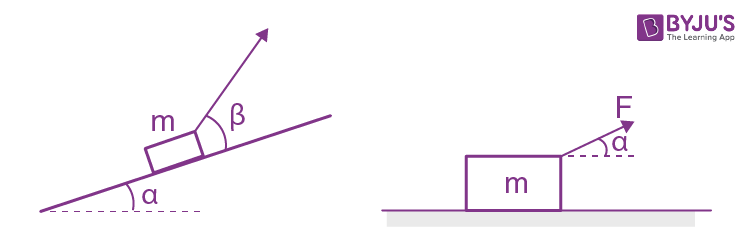1. (a) the velocity of the body at the moment of its breaking off the plane;

2. (b) the distance traversed by the body up to this moment.

Solution:

1. First of all let us draw the free body diagram for the small body of mass m and indicate x-axis along the horizontal plane and y-axis, perpendicular to it, as shown in the figure. Let the block breaks off the plane at t = t0 i.e. N = 0

So N = mg - at0 sin α = 0

Or t0 = mg/a sin α (1)

From Fx = mwx, for the body under investigation: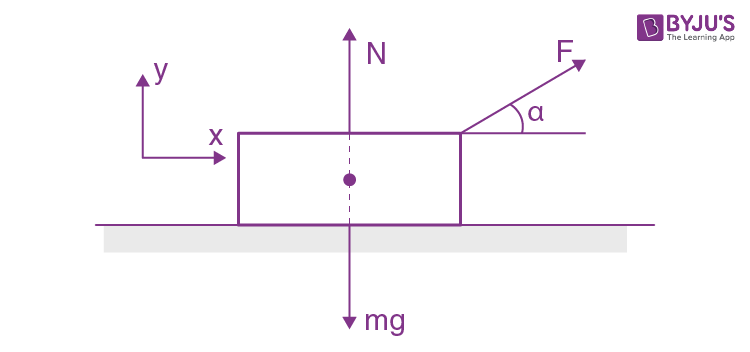mdvx/dt = at cos α; Integrating within the limits for v(t)

m ∫0vdvx = a cos α∫t dt (using (1))

So v = ds/dt = a cos α t2/2m (2)

Integrating Eqn (2) for s(t)

s = (a cos α/2m)(t3/3)

Using the value of t = t0 from Eq. (1) into Eqs. (2) and (3)

v = mg2cos α/2a sin2α

And s = m2g3cos α/6a2 sin3α

11. A bar of mass m resting on a smooth horizontal plane starts moving due to the force F = mg/3 of constant magnitude. In the process of its rectilinear motion, the angle α between the direction of this force and the horizontal varies as α = as, where a is a constant, and s is the distance traversed by the bar from its initial position. Find the velocity of the bar as a function of the angle α.

Solution:

1. Newton’s second law of motion in projection form, along horizontal or x-axis i.e

Fx = mwx gives

F cos (as) = mv dv/ds (as α = as)

Or F cos (as)ds = mv dv

Integrating, over the limits for v(s)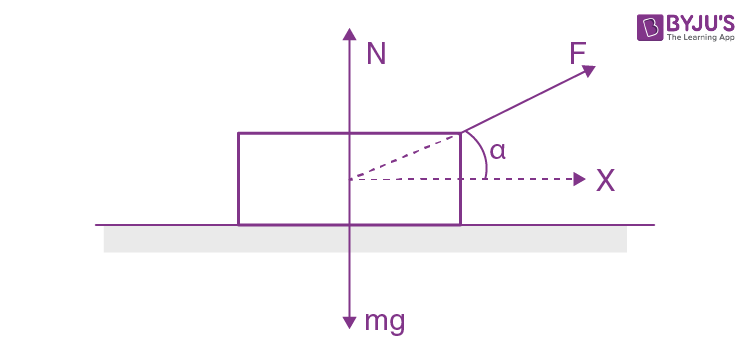$\frac{F}{m}\int_{0}^{\infty }\cos as\: ds=\frac{v^{2}}{2}$

Or v = √(2F sin α/ma)

= √(2g sin α/3a (using F = mg/3) which is the sought relationship.

12. A horizontal plane with the coefficient of friction k supports two bodies: a bar and an electric motor with a battery on a block. A thread attached to the bar is wound on the shaft of the electric motor. The distance between the bar and the electric motor is equal to l. When the motor is switched on, the bar, whose mass is twice as great as that of the other body, starts moving with a constant acceleration w. How soon will the bodies collide?

Solution:

1. From the Newton’s second law in projection from :

For the bar,

T - 2 kmg = (2 m) w ..(1)

For the motor,

T - kmg = m w’ .. (2)

Now, from the equation of kinematics in the frame of bar or motor

l = ½ (w + w’)t2 ..(3)

From (1), (2) and (3) we get on eliminating T and w’

t = √2l/(kg + 3w)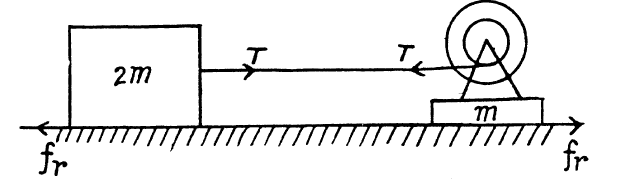13. Find the acceleration w of body 2 in the arrangement shown in Fig. 1.15, if its mass is η times as great as the mass of bar l and the angle that the inclined plane forms with the horizontal is equal to α. The masses of the pulleys and the threads, as well as the friction, are assumed to be negligible. Look into possible cases.

Solution:

1. Let us write Newton’s second law for both, bar 1 and body 2 in terms of projection having taken the positive direction of x1 and x2 as shown in the figure and assuming that body 2 starts sliding, say, upward along the incline

T1 - m1g sin α = m1w1 (1)

m2g - T2 = m2w (2)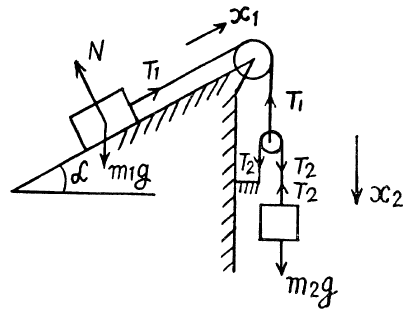For the pulley, moving in vertical direction

from the equation Fx = mwx

2T2 - T1 = (mp)w1 = 0

(as mass of the pulley mp = 0 )

Or T1 = 2T2 ..(3)

As the length of the threads are constant, the kinematical relationship of accelerations becomes w = 2w1 ..(4)

Simultaneous solutions of all these equations yields:

w = 2g(2m2/m1 - sin α)/(4m2/m1 + 1)

= 2g(2η - sin α)/(4η + 1)

As η > 1, w is directed vertically downward, and hence in vector form

$\vec{w}= \frac{2\vec{g}(2\eta -\sin \alpha )}{4\eta +1}$

14. In the arrangement shown in Fig. 1.16 the bodies have masses m0, m1, m2, the friction is absent, the masses of the pulleys and the threads are negligible. Find the acceleration of the body m1. Look into possible cases.

Solution:

1. Let us write Newton’s second law for masses m1 and m2 and moving pully in vertical direction along positive x - axis (Fig.) :

m1g - T = m1w1x (1)

m2g - T = m2w2x (2)

T1 - 2T = 0 (as m = 0)

T1 = 2T (3)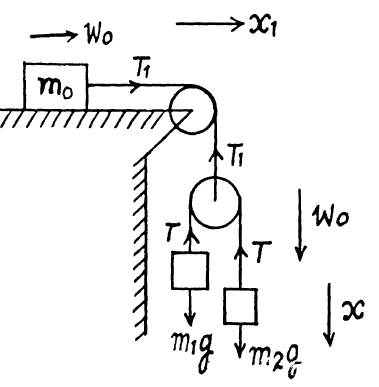Again using Newton’s second law in projection form for mass m0 along positive x1 direction (Fig.), we get

T1 = m0 w0 (4)

The kinematical relationship between the accelerations of masses gives in terms of projection on the x-axis

w1x + w2x = 2w0 (5)

Simultaneous solution of the obtained five equations yields:

w1 = [4m1m2 +m0(m1 - m2)]g/4m1m2 + m0(m1 + m2)

In vector form

$\vec{w_{1}}= \frac{4m_{1}m_{2}+m_{0}(m_{1}-m_{2})}{4m_{1}m_{2}+m_{0}(m_{1}+m_{2})}\vec{g}$

15. In the arrangement shown in Fig. 1.17, the mass of the rod M exceeds the mass m of the ball. The ball has an opening permitting it to slide along the thread with some friction. The mass of the pulley and the friction in its axle are negligible. At the initial moment, the ball was located opposite the lower end of the rod. When set free, both bodies began moving with constant accelerations. Find the friction force between the ball and the thread if t seconds after the beginning of motion the ball got opposite the upper end of the rod. The rod length equals l.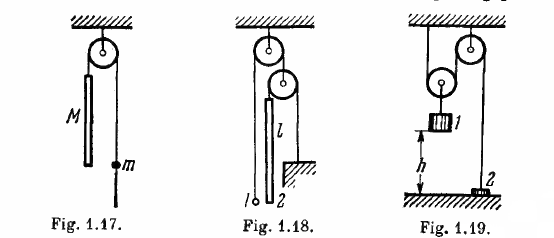Solution:

1. As the thread is not tied with m, so if there were no friction between the thread and the ball m, the tension in the thread would be zero and as a result both bodies will have free fall motion. Obviously, in the given problem, it is the friction force exerted by the ball on the thread, which becomes the tension in the thread. From the condition or language of the problem wM > wm and as both are directed downward so, relative acceleration of M = wM - wm and is directed downward. Kinematical equation for the ball in the frame of rod in projection form along upward direction gives :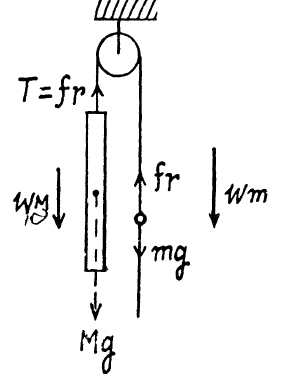l = ½ (wM - wm)t2 (1)

Newton’s second law in projection form along vertically down direction for both, rod and ball gives,

Mg - fr = MwM (2)

mg - fr = mwm (3)

Multiplying Eq. (2) by m and Eq. (3) by M and then subtracting Eq. (3) from (2) and after using Eq. (1) we get

fr = 2lMm/(M - m)t2

16. In the arrangement shown in Fig. 1.18 the mass of ball 1 is η = 1.8 times as great as that of rod 2. The length of the latter is l = 100 cm. The masses of the pulleys and the threads, as well as the friction, are negligible. The ball is set on the same level as the lower end of the rod and then released. How soon will the ball be opposite the upper end of the rod?

Solution:

1. Suppose, the ball goes up with acceleration w1 and the rod comes down with the acceleration w2.

As the length of the thread is constant, 2w1 = w2 ..(1)

From Newton's second law in projection form along vertically upward for the ball and vertically downward for the rod respectively gives,

T - mg = mw1 (2)

And Mg - T’ = Mw2 (3)

but T = 2T (because pulley is massless) (4)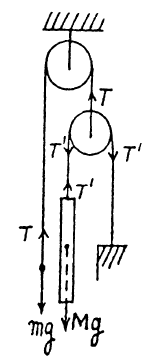From Eqs. (1), (2), (3) and (4)

w1 = (2M - m)g/(m + 4M)

= (2- η)g/(η+4) (in upward direction)

And w2 = 2(2 - η)g/(η+4) (downwards)

From kinematical equation in projection form, we get

l = ½ (w1 + w2)t2

as, w1 and w2 are in the opposite direction. Putting the values of w1 and w2, the sought time becomes

t = √(2l(η +4)/3(2 - η)g) = 1.4s

17. In the arrangement shown in Fig. 1.19 the mass of body 1 is η = 4.0 times as great as that of body 2. The height h = 20 cm. The masses of the pulleys and the threads, as well as the friction, are negligible. At a certain moment body 2 is released and the arrangement set in motion. What is the maximum height that body 2 will go up to?

Solution:

1. Using Newton's second law in projection form along x - axis for body 1 and along negative x-axis for body 2 respectively, we get

m1g - T1 = m1w1 (1)

T2 - m2g = m2w2 (2)

For the pulley lowering in the downward direction from Newton's law along the x-axis,

T1 - 2T2 = 0 (as pulley is massless)

Or T1 = 2T2 (3)

As the length of the thread is constant so

w2 = 2w1 (4)

The simultaneous solution of above equations yields,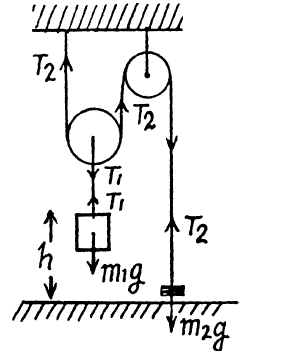w2 = 2(m1 - 2m2)g/(4m2 + m1)

= 2(η -2)/(η + 4) (as m1/m2 = η)

Obviously, during the time interval in which the body 1 comes to the horizontal floor covering the distance h, body 2 moves upward the distance 2h. At the moment when body 2 is at the height 2h from the floor its velocity is given by the expression :

V22 = 2w2(2h) = 2(2(η-2)2gh/(η+4)

= 8h(η-2)g/(η+4)

After the body m1 touches the floor the thread becomes slack or the tension in the thread zero, thus as a result body 2 is only under gravity for it's subsequent motion. Owing to the velocity v2 at that moment or at the height 2h from the floor, the body 2 further goes up under gravity by the distance,

h’ = v22/2g = 4h(η-2)/(η+4)

Thus the sought maximum height attained by the body 2 :

H = 2h + h’ = 2h + 4h(η-2)/(η+4)

= 6ηh/(η+4)

18. Find the accelerations of rod A and wedge B in the arrangement shown in Fig. 1.20 if the ratio of the mass of the wedge to that of the rod equals η, and the friction between all contact surfaces is negligible.

Solution:

1. Let us draw free body diagram of each body, i.e. of rod A and of wedge B, and also draw the kinematical diagram for accelerations, after analyzing the directions of motion of A and B. Kinematical relationship of accelerations is :

tan α = wA/wB

Let us write Newton’s second law for both bodies in terms of projections having taken positive directions of y and x-axes as shown in the figure.

mAg - N cos α = mAwA (2)

And N sin α = mBwB (3)

Simultaneous solution of (1), (2) and (3) yields :

wA = mAg sin α/(mA sin α + mB cot α cos α) = g/(1 + η cot2 α) and

wB = wA/tan α = g/(tan α + η cot α)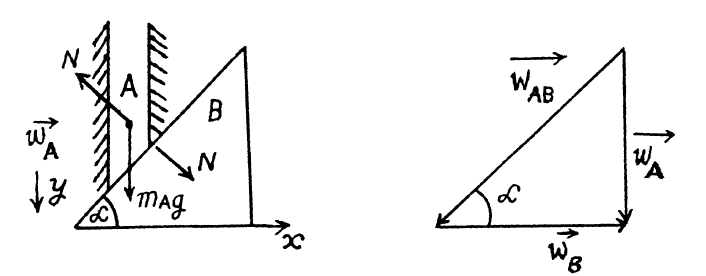Note : We may also solve this problem using conservation of mechanical energy instead of Newton's second law.

19. In the arrangement shown in Fig. 1.21 the masses of the wedge M and the body m are known. The appreciable friction exists only between the wedge and the body m, the friction coefficient being equal to k. The masses of the pulley and the thread are negligible. Find the acceleration of the body m relative to the horizontal surface on which the wedge slides.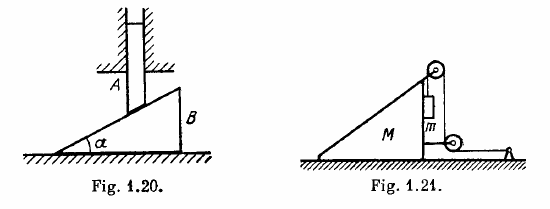Solution:

1. Let us draw free body diagram of each body and fix the coordinate system, as shown in the figure. After analysing the motion of M and m on the basis of force diagrams, let us draw the kinematical diagram for accelerations (Fig.).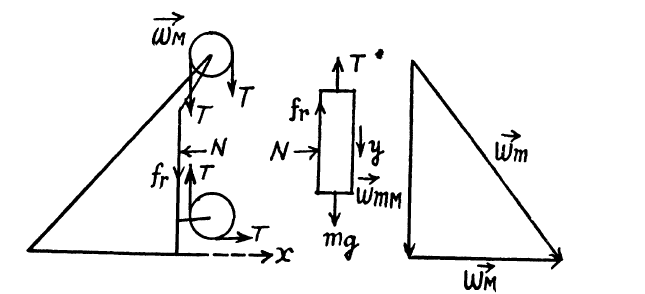As the length of threads are constant so,

dsmM = dsM and as $\vec{v}_{mM}$ and $\vec{v}_{M}$ do not change their directions that why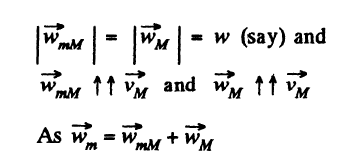So from the triangle law of vector addition

wm = √2w (1)

From the Eq. Fx = mwx , for the wedge and block :

T - N = Mw, (2)

and N = mw (3)

Now, from the Eq. Fy = mwy , for the block

mg - T - kN= mw (4)

Simultaneous solution of Eqs. (2), (3) and (4) yields :

w = mg/(km + 2m + M)

= g/(k + 2 + M/m)

Hence using Eq. 1

wm = g√2/(2 + k + M/m)

20. What is the minimum acceleration with which bar A (Fig. 1.22) should be shifted horizontally to keep bodies 1 and 2 stationary relative to the bar? The masses of the bodies are equal, and the coefficient of friction between the bar and the bodies is equal to k. The masses of the pulley and the threads are negligible, the friction in the pulley is absent.

Solution:

1. Bodies 1 and 2 will remain at rest with respect to bar A for wmin ≤ w ≤ wmax, where wmin is the sought minimum acceleration of the bar. Beyond these limits, there will be a relative motion between bar and the bodies. For 0 ≤ w≤ wmin, the tendency of body 1 in relation to the bar A is to move towards right and is in the opposite sense for w ≥ wmax. On the basis of the above argument the static friction on 2 by A is directed upward and on 1 by A is directed towards left for the purpose of calculating wmin.

Let us write Newton’s second law for bodies 1 and 2 in terms of projection along positive x-axis (Fig.).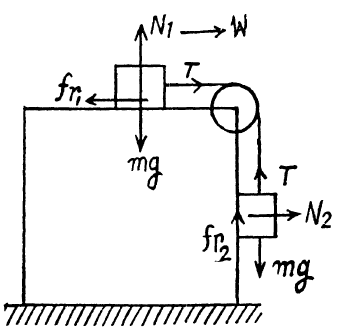T-fr1 = mw or fr1 = T - mw (1)

N2 = mw (2)

As body 2 has no acceleration in vertical direction, so

fr2 = mg - T (3)

From (1) and (3)

(fr1 + fr2) = m(g - w) (4)

But fr1 + fr2 ≤ k(N1 + N2)

Or fr1 + fr2 ≤ k(mg + mw) (5)

From (4) and (5)

m(g - w) ≤ mk(g + w)

Or w ≥ g(1-k)/(1+k)

wmin = g(1-k)/(1+k)